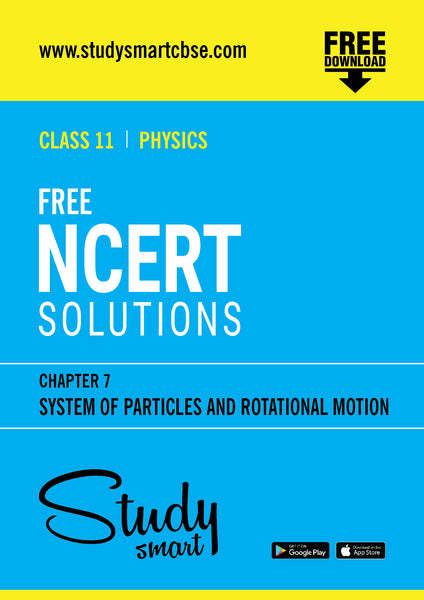# Free NCERT Solutions Class 11th Physics Chapter 7 System of Particles and Rotational Motion

This will be sent as a link to your registered email id

#### Description:

Download Free NCERT Solutions Class 11th Physics Chapter 7 System of Particles and Rotational Motion

Centre of mass of a two-particle system, momentum conservation and centre of mass motion.

Centre of mass of a rigid body; centre of mass of a uniform rod.

Moment of a force, torque, angular momentum, laws of conservation of angular momentum and its applications.

Equilibrium of rigid bodies, rigid body rotation and equations of rotational motion, comparison of linear and rotational motions.

Moment of inertia, radius of gyration, values of moments of inertia for simple geometrical objects (no derivation). Statement of parallel and perpendicular axes theorems and their applications.